### Home > CALC > Chapter 12 > Lesson 12.3.1 > Problem12-112

12-112.
1. The position of a particle moving in the xy-plane has the following parametric equations: x(t) =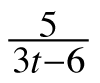, y(t) =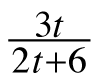Homework Help ✎

1. Determine the slope of the line tangent to the path of the particle when t = 3.

2. Write and interpret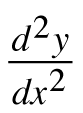.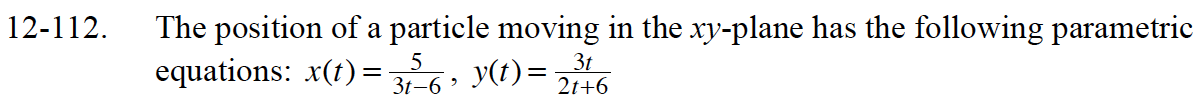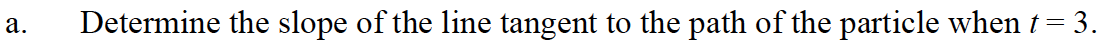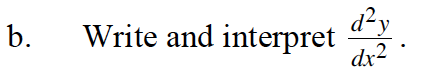$\frac{d^2y}{dx^2}=\frac{d}{dx}\Big(\frac{dy}{dx}\Big)=\frac{d}{dx}\Big(\frac{y^\prime(t)}{x^\prime(t)}\Big)=\frac{dt}{dx}\cdot\frac{d}{dt}\Big(\frac{y^\prime(t)}{x^\prime(t)}\Big)=\frac{\frac{d}{dt}\Big(\frac{y^\prime(t)}{x^\prime(t)}\Big)}{\frac{dx}{dt}}$